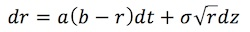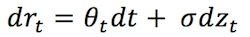### Seeing is believing!

Before you order, simply sign up for a free user account and in seconds you'll be experiencing the best in CFA exam preparation.

##### Subject 6. Term Structure Models
Modern finance seeks to provide models for the shape of the yield curve and the use of the yield curve to value bonds (including those with embedded options) and bond-related derivatives. General equilibrium and arbitrage-free models are the two major types of such models.

General equilibrium term structure models seek to describe the dynamics of the term structure using fundamental economic variables that are assumed to affect interest rates. These models share several characteristics:

• They are one-factor or multi-factor models.
• The make assumptions about the behavior of factors.
• They are, in general, more sparing with respect to the number of parameters that must be estimated compared with arbitrage-free term structure models.

The Cox-Ingersoll-Ross Model

It is a mathematical formula used to model interest rate movements driven by a sole source of market risk.The CIR model believes that short-term interest rates can be represented via a square root diffusion model with a mean reversion. The model is often used in the valuation of interest rate derivatives.

The Vasicek Model

This method of modeling interest rate movement describes the movement of an interest rate as a factor of market risk, time and equilibrium value that the rate tends to revert towards. This stochastic model is often used in the valuation of interest rate futures.

The Vasicek interest rate model values the instantaneous interest rate using the following equation:Where dz is the random market risk and t represents time
a(b-r)dt represents the expected change in the interest rate at t (drift factor)
a is the speed of reversion
b is the long-term level of the mean: All future trajectories of r will evolve around a mean level b in the long run.
σ is the volatility at the time

This model states that the movement of interest rates is affected only by random market movements. In the absence of market shocks (i.e., when σ dz = 0) the interest rate remains constant (rt = b). When rt < b, the drift factor becomes positive, indicating that the interest rate will increase towards equilibrium.

Arbitrage-Free Models: The Ho-Lee Model

The desirability of matching market prices is the central issue in deciding between arbitrage?free and equilibrium models.

An arbitrage-free model of the term structure can be made to fit precisely with the current, observed term structure, so that observed bond yields are equal to the bond yields calculated by the model.

The Ho-Lee Model specifies arbitrage-free movements of flow risk drivers to value a broad range of financial instruments. It uses two parameters: the standard deviation of the short rate, and the risk premium of the short rate.The model can be calibrated to market data by implying the form of θt from market prices, meaning that it can exactly return the price of bonds comprising the yield curve.

User Comment
cfaexaminee Cox-Ingersoll-Ross Model: Assumes the economy has a natural long-run interest rate (b) and the short-term rate (r) converges to.

Vasicek Model: Similar to the CIR model, but assumes that interest rate volatility level is independent of the level of short-term interest rates.

Ho-Lee Model: Calibrated by using market prices to find the time-dependent drift term 0(t) that generates the current term structure.